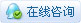1725浏览

# [uPyCraft IDE] ESP32 3.2.4 I2C

I2C是设备之间的两线通信协议。在物理层它只需要两个信号线：SCL 和 SDA，分别是时钟和数据线。I2C 对象关联到总线，它可以在创建时初始化，也可以稍后初始化。

# 类

### class machine.I2C(scl, sda, freq)

``````scl：i2c设备时钟引脚对象
Pin(0)、Pin(2)、Pin(4)、Pin(5)、Pin(9)、Pin(16~19)、Pin(21~23)、Pin(25~27)
sda：i2c设备数据线引脚对象
Pin(0)、Pin(2)、Pin(4)、Pin(5)、Pin(9)、Pin(16~19)、Pin(21~23)、Pin(25~27)
freq：SCL时钟频率
0 < freq ≤ 500000(Hz)``````

### 定义I2C

``````from machine import I2C, Pin

i2c = I2C(scl=Pin(22), sda=Pin(21), freq=10000)``````

## 类函数

### 1. I2C.init(scl, sda, freq)

``````scl：SCL信号线的I/O口
sda：SDA信号线的I/O口
freq：SCL时钟频率``````

``````
i2c.init(scl=Pin(22),sda=Pin(21))``````

### 2. I2C.scan()

``````
i2c.scan()``````

### 3. I2C.start()

``````
i2c.start()``````

### 4. I2C.stop()

``````
i2c.stop()``````

### 5. I2C.write(buf)

``````
buf：存储数据的缓冲区``````

``````buf = b'123'
i2c.start()
i2c.write(buf)``````

``````
buf：存储数据的缓冲区``````

``````buf=bytearray(3)

## 其他类函数

### 标准总线操作

``````addr：i2c设备地址（可由scan函数读取出来）
nbytes：要读取数据的大小``````

``````>>> print(i2c.scan())

>>> print(data)
b'\x00\x02\x00\x00\xe8\x03\xe8\x03'``````

``````addr：i2c设备地址（可由scan函数读取出来）
buf：存储数据的缓冲区``````

``````>>> buf = bytearray(8)
>>> print(buf)
bytearray(b'\x00\x02\x00\x00\xe8\x03\xe8\x03')``````

``````addr：i2c设备地址（可由scan函数读取出来）
buf：存储数据的缓冲区``````

``````>>> b = bytearray(3)
>>> b = 24
>>> b = 111
>>> b = 107
>>> i = i2c.writeto(24,b)
3``````

## 内存操作

``````addr：i2c设备地址（可由scan函数读取出来）
nbytes：要读取的字节大小

``````b = i2c. readfrom_mem(24,  0x58, 3)
print(b)``````

``````
b'\x00\x02\x01'``````

``````addr：i2c设备地址（可由scan函数读取出来）
buf：存储数据的缓冲区

``````buf=bytearray(8)

``````addr：i2c设备地址（可由scan函数读取出来）
buf：存储数据的缓冲区

``````buf = b'123'
i2c.writeto_mem(24, 0x58, buf)``````

# 综合示例

``````from machine import I2C, Pin

i2c0 = I2C(scl=Pin(22), sda=Pin(21), freq=100000)